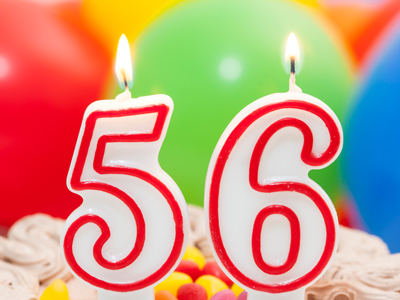The number 56 contains 5 tens and 6 ones.

# Year 2 Numbers - Place Value and Partitioning

This quiz addresses the requirements of the National Curriculum KS1 Maths and Numeracy for children aged 6 and 7 in year 2. Specifically this quiz is aimed at the section dealing with recognising the place value of each digit in a two-digit number (tens, ones) and partitioning numbers in different ways.

Partitioning numbers means being able to recognise the value of each digit within a number given by its place, and separate or 'split' the number into its component parts. For example, 23 could be partitioned into place values of 20 and 3 (2 tens and 3 ones), and 456 could be partitioned into 400, 50 and 6 (4 hundreds, 5 tens and 6 ones). Partitioning can also be done in different ways - for example, 23 could be partitioned into 13 and 10.

Try this quiz to see how well you do with partitioning numbers.

Question 1
How could you partition 47?
14 + 17
4 + 7
70 + 4
40 + 7
47 is 4 tens and 7 ones
Question 2
How could you partition 56?
15 + 16
5 + 6
50 + 60
20 + 36
The 5 tens in 56 could be split into 20 and 30
Question 3
What is 78 - 20?
28
55
76
58
There are 7 tens in 78 - taking away 2 of them leaves 5
Question 4
What is the value of the 3 in 35?
3
30
300
33
35 can be partitioned into 30 and 5
Question 5
How could you partition 18?
1 + 8
10 + 8
80 + 1
18 + 8
18 is a ten and 8 ones
Question 6
How could you partition 25?
10 + 10
50 + 2
20 + 5
2 + 5
25 is 2 tens and 5 ones
Question 7
What is 69 - 30?
63
66
39
36
There are 6 tens in 69 - taking away 3 of them leaves 3
Question 8
What is the value of the 6 in 46?
6
60
16
600
46 can be partitioned into 4 tens and 6 ones
Question 9
How could you partition 28?
2 + 8
10 + 18
20 + 80
10 + 28
There are 2 tens in 28
Question 10
How could you partition 46?
10 + 10 + 10 + 10 + 6
4 + 6
40 + 6 + 6
4 + 4 + 4 + 6
There are 4 tens in 46
Author:  Angela Smith